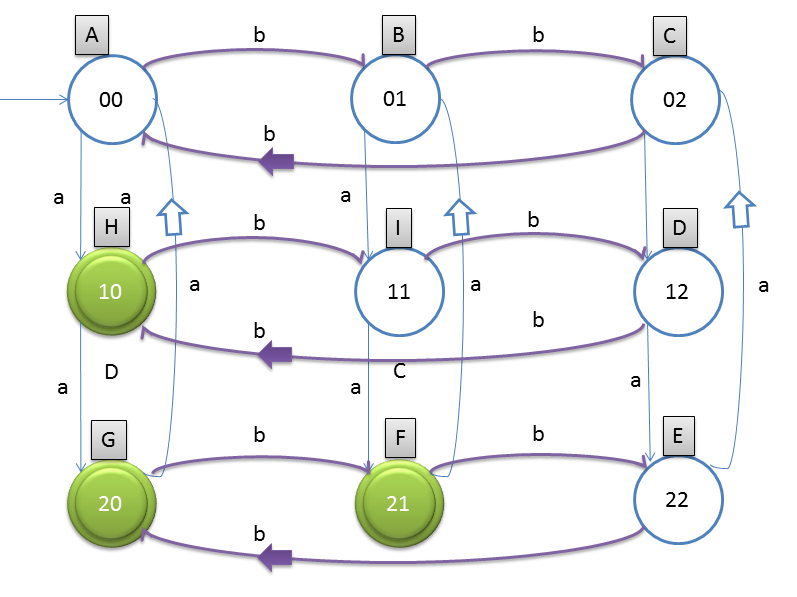# DFA String Examples

Design a DFA such a way that
Let string is w, Number of a(w) mod 3 > Number of b(w) mod 3 This means (number of a divisible by 3) should be greater than (number of b divisible by 3).

Final states = {H, G, F}